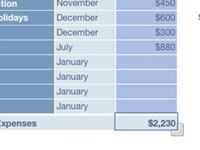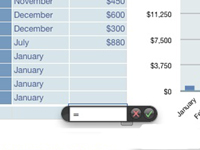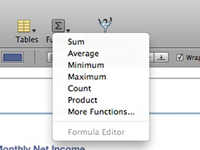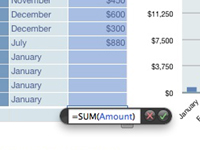How to Create Formulas in a Snow Leopard Numbers Spreadsheet - dummies

# How to Create Formulas in a Snow Leopard Numbers Spreadsheet

In Snow Leopard’s Numbers application you use formulas — equations that calculate values based on the contents of cells you specify in your spreadsheet. For example, if you designate cell A1 of a Numbers spreadsheet (the cell in column A at row 1) to hold your yearly salary and cell B1 to hold the number 12, you can divide the contents of cell A1 by cell B1 (to calculate your monthly salary) by typing this formula into any other cell:

`=A1/B1`

Formulas in Numbers always start with an equal sign (=).

You can use a calculator, but formulas are much easier. For example, say you want to calculate your weekly salary. Rather than grab a pencil and paper, you can simply change the contents of cell B1 to 52, and the spreadsheet is updated to display your weekly salary.

That’s a simple example, of course, but it demonstrates the basis of using formulas (and the reason that spreadsheets are often used to predict trends and forecast budgets).## 1Select the cell.

Choose the cell that will hold the result of your calculation.## 2Click inside the Formula Box and type = (the equal sign).

The Formula Box appears to the right of the Sheets heading, directly under the Button bar. Note that the Format Bar changes to show a set of formula controls (aka the Formula Bar).## 3Click the Function Browser button.

The button bears the fx label and appears next to the red Cancel button on the Formula Bar.## 4Click the desired formula and click Accept.

Your formula is added to the Formula box.